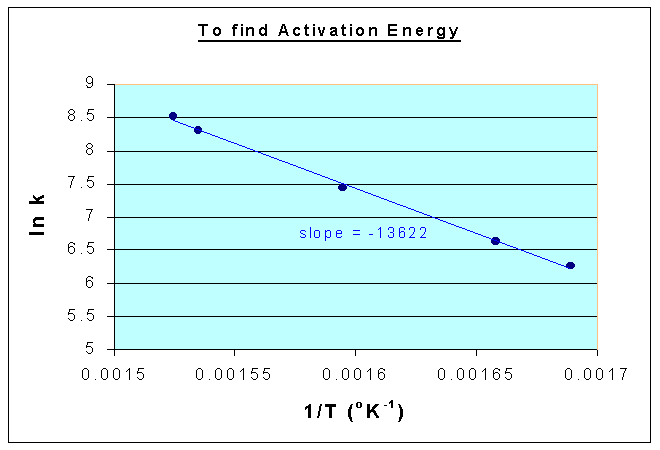### Activation Energy

The decomposition of NO2 follows a second order rate equation. Data at different temperatures are as follows:

```T (K)		592	603	627	651.5	656
k (cm3/gmol.sec)  522	755	1700	4020	5030```

Compute the energy of activation Energy from the data. The reaction is 2NO2 → 2NO + O2

Calculations:

Activation energy is found from the Arrhenius' relation

k = ko e-E/RT

i.e.,

ln k vs. 1/T is a straight line with a slope of -E/R

where, E is the activation energy; and

R is the universal gas constant.

 T (K) 592 603 627 651.5 656 k (cm3/gmol.sec) 522 755 1700 4020 5030 1/T (oK-1) 0.001689 0.001658 0.001595 0.001535 0.001524 ln k 6.25767 6.62672 7.43838 8.29904 8.52318

From the above data, the following graph is drawn, and the slope is = -13622Therefore,

E/R = 13622

E = 13622 x 8314 = 113253.3 kJ/kmol